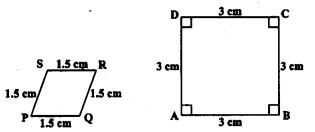KSEEB SSLC Class 10 Maths Solutions Chapter 2 Triangles Ex 2.1

In this chapter, we provide KSEEB SSLC Class 10 Maths Solutions Chapter 2 Triangles Ex 2.1 for English medium students, Which will very helpful for every student in their exams. Students can download the latest KSEEB SSLC Class 10 Maths Solutions Chapter 2 Triangles Ex 2.1 pdf, free KSEEB SSLC Class 10 Maths Solutions Chapter 2 Triangles Ex 2.1 book pdf download. Now you will get step by step solution to each question.

Karnataka State Syllabus Class 10 Maths Chapter 2 Triangles Ex 2.1

Question 1.
Fill In the b1ank using the correct word given in brackets:
i) All circles are _______ . (congruent, similar)
Solution:
congruent

ii) All squares are _______. (similar, congruent)
Solution:
similar

iii) All ______ triangles are similar. (isosceles, equilateral)
Solution:
equilateral

iv) Two polygons of the same number of sides are similar, if
(a) their corresponding angles are _______ and
(b) their corresponding sides are _______. (equal, proportional).
Solution:
(a) equal
(b) proportional

Question 2.
Give two different examples of pair of
i) similar figures
Solution:
coin, wheel of a cart.

ii) non-similar figures.
Solution:
A square Rhombus

Question 3.
State whether the following quadrilaterals are similar or not:Solution:
Here, two diagrams PQRS and ABCD are rhombus and square whose sides are in proportional. But the corresponding angle is not equal. Hence these are not similar.

All Chapter KSEEB Solutions For Class 10 Maths

—————————————————————————–

All Subject KSEEB Solutions For Class 10

*************************************************

I think you got complete solutions for this chapter. If You have any queries regarding this chapter, please comment on the below section our subject teacher will answer you. We tried our best to give complete solutions so you got good marks in your exam.

If these solutions have helped you, you can also share kseebsolutionsfor.com to your friends.

Best of Luck!!